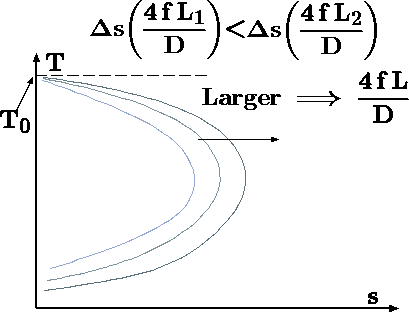Fig. 11.23 The effects of increase of $$\dfrac{4\,f\,L}{D}$$ on the Fanno line.
It has to be recognized that there are two regimes that can occur in Fanno flow model one of subsonic flow and the other supersonic flow. Even the flow in the tube starts as a supersonic in parts of the tube can be transformed into the subsonic branch. A shock wave can occur and some portions of the tube will be in a subsonic flow pattern. The discussion has to differentiate between two ways of feeding the tube: converging nozzle or a converging-diverging nozzle. Three parameters, the dimensionless friction, $$\dfrac{4\,f\,L}{D}$$, the entrance Mach number, $$M_1$$, and the pressure ratio, $$P_2/P_1$$ are controlling the flow. Only a combination of these two parameters is truly independent. However, all the three parameters can be varied and some are discussed separately here.